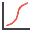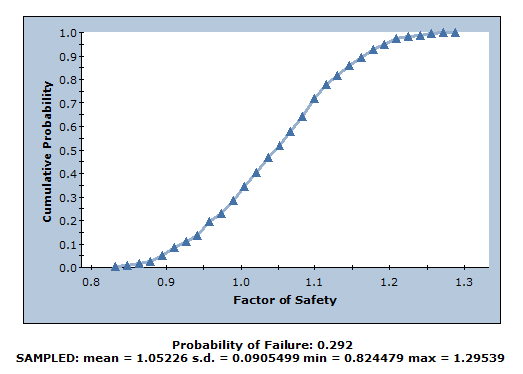The Rocscience International Conference 2021 Proceedings are now available. Read Now

# Cumulative Plot

To plot a cumulative distribution after a Probabilistic analysis:

1. Select Cumulative Ploton the toolbar or the Statistics menu to open the Cumulative Plot dialog.2. Select the data you want to plot in the Data to Plot (e.g., Factor of Safety or one of the parameters you've defined as a random variable).
3. Enter a Number of Bins. The default is 30, but any value between 2 and 100 can be entered.
4. Click Plot.
The cumulative distribution is generated in the Cumulative Plot View, and the mean, standard deviation, minimum and maximum values for the data plotted are displayed at the bottom.

A Cumulative Distribution is, mathematically speaking, the integral of the normalized probability density function. Practically speaking, a point on the cumulative distribution gives us the probability that a random variable will be LESS THAN OR EQUAL TO a specified value.

That is, if (X , Y) is a point on the cumulative distribution S-curve, then Y = the probability that the random variable will be <= X.

The data Sampler allows you to obtain the coordinates of points on the S-curve.

The same Data Types which can be plotted on a Histogram can also be plotted as a Cumulative Distribution.

Cumulative plot of safety factor distribution## Right Click Options

A variety of plotting and export options are available in the Cumulative Plot View right-click menu. These include:

• Vertex Markers
• Sampler
• Chart Properties
• Change Plot Data
• Copy
• Copy Data
• Plot in Excel
• Export Image